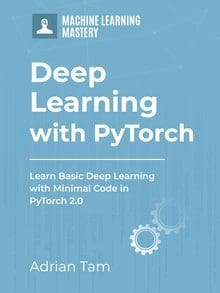# Making Predictions with Logistic Regression in PyTorch

Logistic regression is a statistical technique for modeling the probability of an event. It is often used in machine learning for making predictions. We apply logistic regression when a categorical outcome needs to be predicted.

In PyTorch, the construction of logistic regression is similar to that of linear regression. They both applied to linear inputs. But logistic regression is specifically classification problems, such as classifying into one of the two outcomes (0 or 1).

In this tutorial, we’ll focus on making predictions with logistic regression. We’ll learn how some of the useful packages in the PyTorch library can help easily create a logistic regression model. Particularly, we’ll learn:

• How to make predictions with logistic regression in PyTorch.
• The logistic function and its implementation on tensors.
• How to build a logistic regression model with nn.Sequential.
• How to build a custom module for logistic regression.

Kick-start your project with my book Deep Learning with PyTorch. It provides self-study tutorials with working code.

Let’s get started.Making Predictions with Logistic Regression in PyTorch.
Picture by Manson Yim. Some rights reserved.

## Overview

This tutorial is in four parts; they are

• Create Data Class
• Build the Model with nn.Module
• Train with Mini-Batch Gradient Descent
• Plot the Progress

## What is a Logistic Function?

When class of a certain point in a dataset is calculated using a linear function, we get a positive or a negative number such as $-3$, $2$, $4$, etc. When we build a classifier, or specifically a binary classifier, we wish it can return either 0 or 1. A sigmoid or logistic function can be used in this case as this function always return a value between 0 and 1. Usually we will set a threshold, such as 0.5, to round up or round down the result to designate the output to one class or another.

In PyTorch, the logistic function is implemented by the nn.Sigmoid() method. Let’s define a tensor by using the range() method in PyTorch and apply the logistic function to observe the output.

Let’s see how the plot looks like.Logistic function

As you can see in the plot, the values of a logistic function range between 0 and 1, with the transition happen mostly around 0.

### Want to Get Started With Deep Learning with PyTorch?

Take my free email crash course now (with sample code).

Click to sign-up and also get a free PDF Ebook version of the course.

## Logistic Regression Model via nn.Sequential

The nn.Sequential package in PyTorch enables us to build logistic regression model just like we can build our linear regression models. We simply need to define a tensor for input and process it through the model.

Let’s define a Logistic Regression model object that takes one-dimensional tensor as input.

This model has a linear function layer. The output from the linear function is passed on to the logistic function that makes the prediction.

We can check the list of model parameters using parameters() method. The parameters should be randomly initialized in this case but we can see the shape match what we specified in the model above.

Here’s what the output looks like.

Now, let’s define a one-dimensional tensor x and make predictions with our logistic regression model.

We force the tensor to be in float32 type because this is what our model expects. Feeding this samples of data into the model, we will get the following predictions.

Its output is like the following:

Putting everything together, the following is the complete code:

## Custom Module for Logistic Regression

Knowing how to build custom modules is necessary when you work on advanced deep learning solutions. We can try out the syntax and build our custom logistic regerssion module. This should work identically to the nn.Sequential model above.

We’ll define the class and inherit all the methods and attributes from the nn.Module package. In the forward() function of the class, we’ll use sigmoid() method which takes the output from the linear function of the class and makes the prediction.

We can instantiate the class object.

Now, let’s make predictions for the tensor x we defined above.

The output would be:

As you can see, our custom model for Logistic Regression works exactly like the nn.Sequential version above.

Putting everything together, the following is the complete code:

## Summary

In this tutorial, you learned some basics of Logistic Regression and how it can be implemented in PyTorch. Particularly, you learned:

• How to make predictions with Logistic Regression in Pytroch.
• About the Logistic Function and its implementation on tensors.
• How to build a Logistic Regression model with nn.Sequential.
• How to build a custom module for Logistic Regression.

## Get Started on Deep Learning with PyTorch!#### Learn how to build deep learning models

...using the newly released PyTorch 2.0 library

Discover how in my new Ebook:
Deep Learning with PyTorch

It provides self-study tutorials with hundreds of working code to turn you from a novice to expert. It equips you with
tensor operation, training, evaluation, hyperparameter optimization, and much more...

### 2 Responses to Making Predictions with Logistic Regression in PyTorch

1.Andrew N December 23, 2022 at 10:59 am #